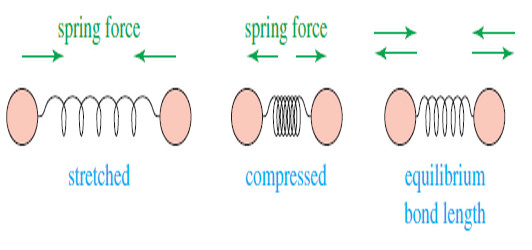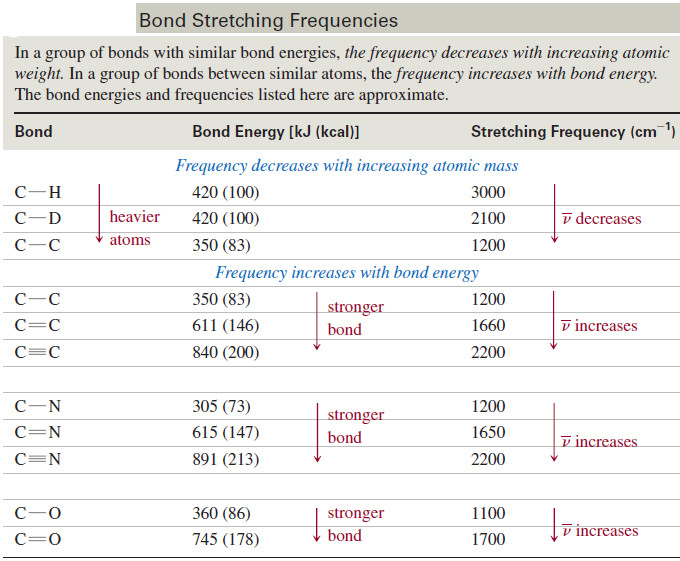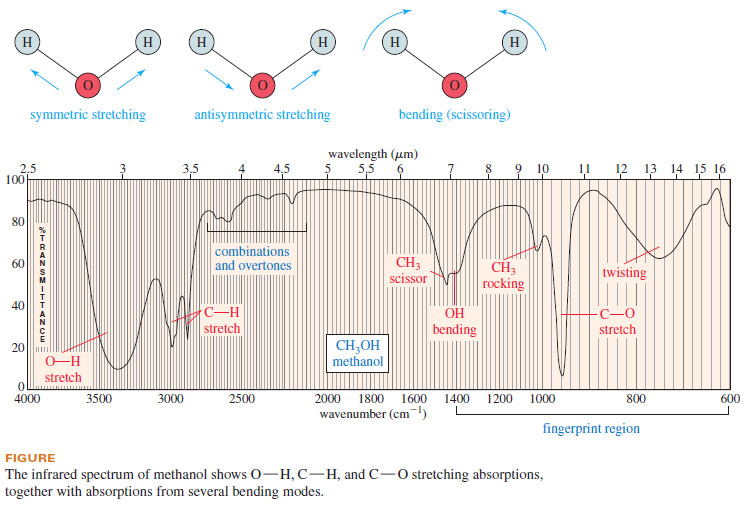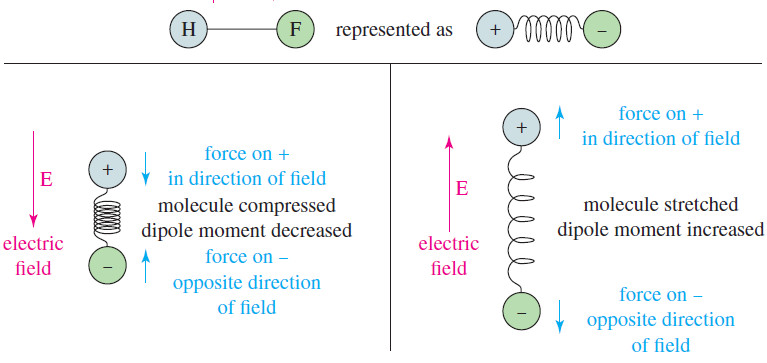# Molecular Vibrations : IR spectrum

– In this subject we talk about Molecular Vibrations as introduction to understand IR spectrum

## Molecular Vibrations

– Before discussing characteristic infrared absorptions, it’s helpful to understand some theory about the vibrational energies of molecules.

– The following drawing shows how a covalent bond between two atoms acts like a spring.

– If the bond is stretched, a restoring force pulls the two atoms together toward their equilibrium bond length.

– If the bond is compressed, the restoring force pushes the two atoms apart.

– And If the bond is stretched or compressed and then released, the atoms vibrate.– The frequency of the stretching vibration depends on the masses of the atoms and the stiffness of the bond.

– Heavier atoms vibrate more slowly than lighter ones; for example, the characteristic frequency of a C-D bond is lower than that of a C-H bond.

– In a group of bonds with similar bond energies, the frequency decreases with increasing atomic weight.

– Stronger bonds are generally stiffer, requiring more force to stretch or compress them.

– Thus, stronger bonds usually vibrate faster than weaker bonds (assuming the atoms have similar masses).

– For example, O-H bonds are stronger than C-H bonds, so O-H bonds vibrate at higher frequencies.

– Triple bonds are stronger than double bonds, so triple bonds vibrate at higher frequencies than double bonds.

– Similarly, double bonds vibrate at higher frequencies than single bonds.

– In a group of bonds having atoms of similar masses, the frequency increases with bond energy.

– The following table lists some common types of bonds, together with their stretching frequencies, to show how frequency varies with the masses of the atoms and the strengths of the bonds.## Infrared spectrum

– An infrared spectrum  (IR spectrum) is a graph of the energy absorbed by a molecule as a function of the frequency or wavelength of light.

– The IR spectrum of methanol is shown in Figure 12-2.

– In the infrared region, absorptions generally result from exciting the vibrational modes of the bonds in the molecule.

– Even with simple compounds, infrared spectra contain many different absorptions, not just one absorption for each bond.

– The methanol spectrum (Figure down) is a good example.

– We can see the broad stretch around 3300 cm-1 ,the C-H stretch just below 3000 cm-1 and the C-O stretch just above 31000 cm-1 .

– We also see absorptions resulting from bending vibrations, including scissoring and twisting vibrations.

– In a bending vibration, the bond lengths stay constant, but the bond angles vibrate about their equilibrium values.

– Consider the fundamental vibrational modes of a water molecule in the following diagram.

– The two O-H bonds can stretch in phase with each other (symmetric stretching), or they can stretch out of phase (antisymmetric stretching).

– The H-O-H bond angle can also change in a bending vibration, making a scissoring motion– A nonlinear molecule with (n) atoms generally has (3n-6) fundamental vibrational modes.

– Water (3 atoms) has 3(3) – 6 = 3 fundamental modes, as shoewn in the preceding figure.

– Methanol has 3(6) – 6 = 12 fundamental modes, and ethanol has 3(9) – 6 = 21 fundamental modes.

– We also observe combinations and multiples (overtones) of these simple fundamental vibrational modes.

– As you can see, the number of absorptions in an infrared spectrum can be quite large, even for simple molecules.

– It is highly unlikely that the IR spectra of two different compounds (except enantiomers) will show the same frequencies for all their various complex vibrations.

– For this reason, the infrared spectrum provides a “fingerprint” of a molecule.

– In fact, the region of the IR spectrum containing most of these complex vibrations (600 to 1400 cm-1)  is commonly called the fingerprint region of the spectrum.

– The simple stretching vibrations in the 1600 to 3500 cm-1 region are the most characteristic and predictable.

– Although our introductory study of IR spectra will largely ignore bending vibrations, you should remember that these absorptions generally appear in the 600 to 1400 cm-1 region of the spectrum.

– Experienced spectroscopists can tell a great deal about the structure of a molecule from the various kinds of bending vibrations known as “wagging,” “scissoring,” “rocking,” and “twisting” that appear in the fingerprint region (see Figure above).

## IR-Active and IR-Inactive Vibrations

– Not all molecular vibrations absorb infrared radiation.

– To understand which ones do and which do not, we need to consider how an electromagnetic field interacts with a molecular bond.

– The key to this interaction lies with the polarity of the bond, measured as its dipole moment.

– A bond with a dipole moment can be visualized as a positive charge and a negative charge separated by a spring.

– If this bond is placed in an electric field (see Figure), it is either stretched or compressed, depending on the direction of the field.– One of the components of an electromagnetic wave is a rapidly reversing electric field (E)

– This field alternately stretches and compresses a polar bond, as shown in Figure.

– When the electric field is in the same direction as the dipole moment, the bond is compressed and its dipole moment decreases.

– When the field is opposite the dipole moment, the bond stretches and its dipole moment increases.

– If this alternate stretching and compressing of the bond occurs at the frequency of the molecule’s natural rate of vibration, energy may be absorbed.

– Vibrations of bonds with dipole moments generally result in IR absorptions and are said to be IR-active.

– If a bond is symmetrical and has zero dipole moment, the electric field does not interact with the bond.

– For example, the triple bond of acetylene  (H-C=C-H) has zero dipole moment, and the dipole moment remains zero if the bond is stretched or compressed.

– Because the vibration produces no change in the dipole moment, there is no absorption of energy.

– This vibration is said to be IR-inactive, and it produces no absorption in the IR spectrum.

– The key to an IR-active vibration is that the vibration must change the dipole moment of the molecule.

– In general, if a bond has a dipole moment, its stretching frequency causes an absorption in the IR spectrum.

– If a bond is symmetrically substituted and has zero dipole moment, its stretching vibration is weak or absent in the spectrum.

– Bonds with zero dipole moments sometimes produce absorptions (usually weak) because molecular collisions, rotations, and vibrations make them unsymmetrical part of the time.

– Strongly polar bonds (C=O groups, for example) may absorb so strongly that they also produce overtone peaks, which are relatively small peaks at a multiple (usually double) of the fundamental vibration frequency